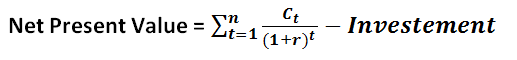# Internal Rate of Return (IRR)

Internal Rate of Return is the rate at which the Net Present Value is Zero. This is among the most used calculation in Capital Budgeting. IRR is also known as Discounted Cash Flow of Return or Economic Rate of Return.

As we know,By Equating the above equation to 0 we get,OR
If IRR > Expected Rate of Return; Project is Profitable
If IRR < Expected Rate of Return; Project is not Profitable
• At IRR, present value of all tax-free future cash flows becomes equal to the initial investment.
• If IRR is greater than the expected rate of return, then the project is profitable.
• For individual project, if the IRR is greater than the expected rate of return, project should be taken.
• For comparing between the projects, the project with highest IRR must be taken if it is greater than the expected rate of return.
• IRR Calculation for an investment is easy if the life cycle of the investment is short, but if the life cycle of the investment is of many years, the IRR is calculated by hit and trial.
Example 1: A project requires an input capital of Rs. 10000.00 and generates the cash flow of Rs. 12000.00 after a year. The required rate of return is 25%. Calculate the profitability of the project.
Solution:
Calculating IRR by substituting NPV = 0(1+IRR) = 12000/10000 = 1.2
IRR = 0.2 or 20%
As, IRR >Expected Rate of Return, hence the project is profitable.